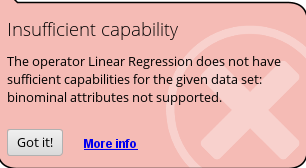# Convert categorical variables into dummy variables

Member Posts: 2Newbie
in Help
Hi, I want to perform a regression task to predict continuous response. I have 4 categorical variables, others are numerical.

Categorical variables are:
age=(≤20, 21-35, 36-50, ≥51)
gender=(Female, Male)
income level=(1=insufficient, 2=sufficient)
BMI range=(1=<25, 2=>25)
*Income level & BMI are keyed in as numerical code in my dataset

Let's say I want to perform SVM, RF, Decision Tree, MLR, and KNN;

1. Should I convert all categorical variables into dummy variables?
2. If using numerical coding is more suitable, should I change the data type to nominal (binominal/polynominal) or retain it as integer?
Tagged:

• Member Posts: 57Guru